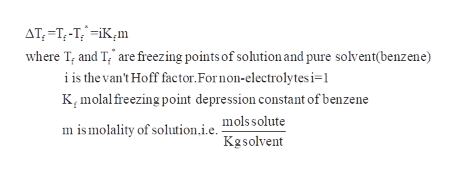# When 271. mg of a certain molecular compound X are dissolved in 25.0g of benzene C6H6, the freezing point of the solution is measured to be 0.5°C. Calculate the molar mass of X. If you need any additional information on benzene, use only what you find in the ALEKS Data resource. Also, be sure your answer has a unit symbol, and is rounded to 2 significant digits.molal freezing point depression constant (Kf)°C·kg·mol−1  of benzene is 5.07

Question
124 views

When 271. mg of a certain molecular compound X are dissolved in 25.0g of benzene C6H6, the freezing point of the solution is measured to be 0.5°C. Calculate the molar mass of X.

If you need any additional information on benzene, use only what you find in the ALEKS Data resource. Also, be sure your answer has a unit symbol, and is rounded to 2 significant digits.

molal freezing point depression constant (Kf)
°C·kg·mol−1  of benzene is 5.07

check_circle

Step 1

Given:

Mass of molecular compound X=271. mg

Mass of benzene=25.0 g

Freezing point of solution=0.5oC

Molal freezing point depression constant(Kf) of benzene=5.07 OC .Kg.mol-1

Conversion:

From mg to g:

Mass of molecular compound X=271. mg

=271/1000=0.271 g

From g to Kg:

Mass of benzene=25.0 g

=25.0/1000=0.025 Kg

Step 2

To calculate molar mass of X(solute):

First we have to find molality, the following equation ishelp_outlineImage TranscriptioncloseAT-T-T;iKm where T, and T, are freezing points of solution and pure solvent(benzene) i is the van't Hoff factor. Fornon-electrolytesi=1 Kmolalfreezing point depression constant of benzene mols solute mismolality of solution.i.e Kgsolvent fullscreen
Step 3

Substitute all the values in the above equation, we get the molal...

### Want to see the full answer?

See Solution

#### Want to see this answer and more?

Solutions are written by subject experts who are available 24/7. Questions are typically answered within 1 hour.*

See Solution
*Response times may vary by subject and question.
Tagged in

### Physical Chemistry